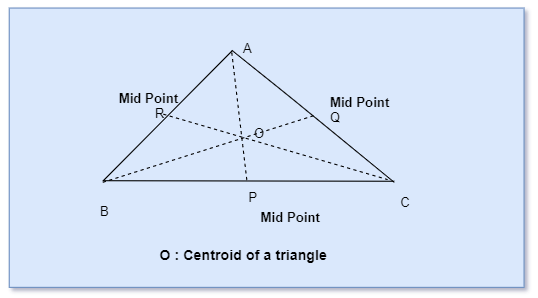# Centroid of a Triangle CalculatorNote
• Enter the values of Coordinates of the triangle
• Click on the calculate button.

Formula And concept used
$x_c = \frac {x_1 + x_2 + x_3}{3}$
$y_c = \frac {y_1 + y_2 + y_3}{3}$

### Centroid of a Triangle Calculator

Example of Few questions where you can use this Centroid of a Triangle Calculator
1. Find the Centroid of a Triangle.
i. A(2,3), B(4 ,5) , C(5,9)
ii. A(-1,3), B(5 ,8) , C(2,2)
iii. A(1,1), B(2 ,5) , C(1,7)
iv. A(2,3), B(5 ,4) , C(9,5)

## Related Calculators

Latest Articles
Synthetic Fibres and Plastics Class 8 Practice questions

Class 8 science chapter 5 extra questions and Answers

Mass Calculator

3 Fraction calculator

Garbage in Garbage out Extra Questions7